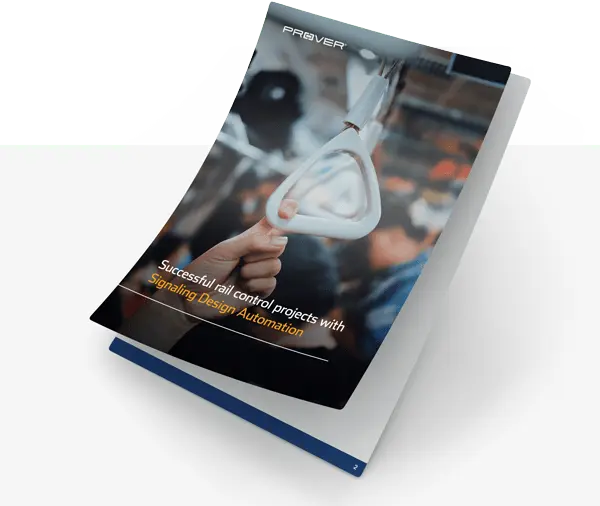In a previous blog post, a playful concrete example was given to show off PSL’s newly added support for IEEE 754 floating point calculations. We have tested the performance in real world industrial examples and in this blog post we will take a look at the floating point performance of PSL on a real world problem from avionics.

### Triple Redundant Sensor Voter

In order to meet high redundancy requirements in avionics, a common technique is to use triple redundant sensor voters. To create a reliable signal, the outputs of three identical sensors are composed into a single signal by a voting component. The voting mechanism averages/centers the set of three input values over time to avoid being overly affected by a single faulty sensor.

The sensor voter model can be thought of as consisting of a set of floating point latches that are updated at every reading. The output is computed from the input of the three sensors and from these latches. If one disregards the floating point rounding, the latches and the output have piecewise linear definitions. The rounding makes the model non-linear.

Depending on the type of sensors (range, measurement uncertainty, etc) a typical voting algorithm needs to be configured by setting different constants(thresholds etc). The resulting behavior of the sensor voter, and checks that it is sufficiently reliable, is traditionally tested by massive simulation, which is very costly. Besides being costly, simulation can only give partial coverage.

Examples of typical requirements on the sensor voter implementation are:

– Given a bound on sensor errors (for non-faulty sensors), the error of the voter output must be sufficiently bounded.

– Given a bound on signal change (between two readings), the change in voter output must be sufficiently bounded.

To get full coverage one can instead of simulation, perform formal verification. One efficient way is to analyze the sensor voter with an SMT solver assuming that the arithmetic operations are exact (no rounding), since in this case the system is piecewise linear. The downside is that then additional argumentation is needed to take into account the “errors” introduced by rounding, i.e. handling the difference between operations on real (mathematical) numbers and operations on floating point numbers (which are rounded).

Instead of working with real numbers, a better and more complete solution would be to precisely model the floating point numbers and their operations(including rounding) when performing formal verification. A stumbling block for this approach has been performance issues. Modeling floating point arithmetic at bit level requires a significant boost in verification tool performance. This is the problem we have addressed when implementing floating point numbers in PSL.

### Excellent Results

In order to really test the performance of floating point arithmetic in PSL we used double precision (64-bit) IEEE 754 floats in the HLL model. Performance often tend to degrade rapidly with increased precision, in particular when models are non-linear.

To reach the required performance boost and to fully utilize modern processor architectures, PSL uses powerful parallelization and machine learning to optimize the proof strategy.

The two requirements mentioned above where proved together five times, one for each rounding mode defined in IEEE 754. The running time on a modern multi-core processor was between 2 and 3 minutes, depending on the rounding mode.

Even though these results are impressive, as mentioned in Floating Point Model Checking, floating point verification is notoriously hard and the performance depends a lot on the complexity of the actual models. Also one needs to be very careful in order to keep the full precision of the verification valid throughout the real system:

– One needs control over possible rewritings/optimizations in the compiler, and

– one needs to make sure that the platform, on which the software is executed, exactly implements the IEEE 754 standard for the functionality which is actually used.

That said, PSL sets a high standard for floating point performance, combining precision with power. It gives the required boost to allow efficient floating point verification.